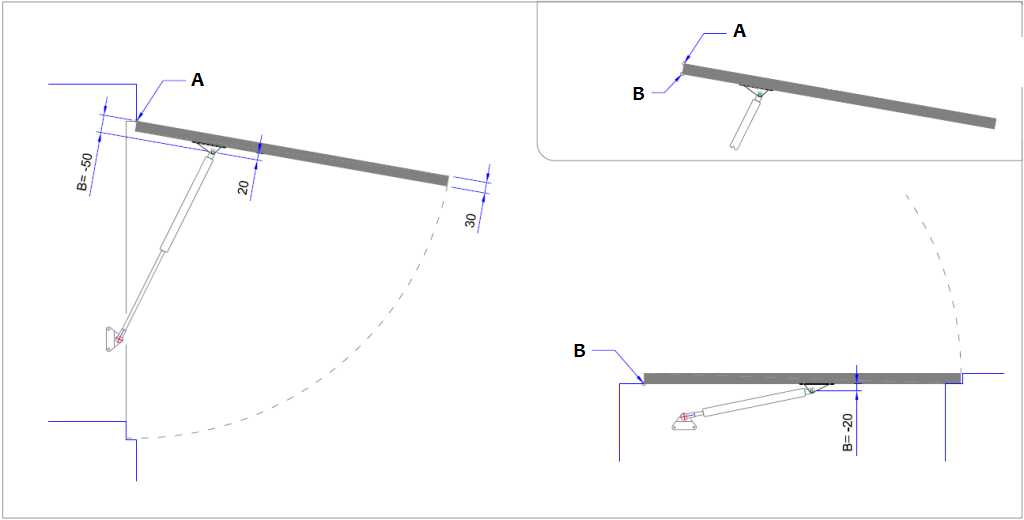# Why is the B measurement important? And how do I change that while maintaining the operation of the gas springs?

In the result of the Calculation tool the B measurement, along with the A measurement, displays the position of the centre of the end fitting of the gas spring to the cover.

Both of these distances will be shown from the centre of the pivot point from the cover.

The A measurement is a distance measured along the cover and the B measurement is a distance measured perpendicular to that.

The A measurement is a value that comes out of the gas spring calculation while the B measurement is directly dependent on the distance between the end fitting of the gas strut and the surface of the cover.

The Calculation tool sets the B measurement on the basis of the entered thickness of the cover and the position of the pivot point relative to the cover with thereby the point of attachment of the gas spring being 20 mm away from the cover surface as standard.

Below are some examples of the B measurement set by the Calculation tool (A = hinge on top, B = hinge on bottom):Once ‘Calculate’ is pressed in in Calculation tool (in Step 2), the B measurement is determined by a standard calculation rule and gas spring application is calculated.

The B measurement, which is then as shown in the result of the calculation, must correspond to the B distance from the point of attachment of the gas spring as it will eventually be mounted on the cover.

It may be that the final B distance from the attachment point of the gas spring is different from the B measurement as calculated as standard by the Calculation tool (A= pivot point):In that case, the B measurement must be adjusted.

The main reason for that is that the attachment of the gas strut to the mounting point, indicated in the calculation tool by the C measurement and D measurement, must geometrically match the retracted and extended gas spring length combined with the rotation of the cover (from ‘closed’ angle to ‘open’ angle).

Before adjusting the B measurement, note the hand forces as they are shown in ‘show open’ and ‘show closed’.

Only then adjust the B measurement and change the A measurement until, in both ‘open’ as well as ‘closed’, the previously noted values for the hand forces are reached again.

Posted in: Calculation